## FPGA学习笔记

2021/5/17 22:59:17 人评论

0 FPGA概述
0.1 Verilog基础语法
1 开发流程
1.1 案例：控制LED灯
1.2 案例：3-8译码器（比较详细）
2 层次化设计
2.1 案例：全加器

# 0 FPGA概述

1. FPGA技术优势
(1) 速度快
(2) 效率高
(3) 低延时
(4) 可重构
(5) 开发灵活方便
(6) 接口丰富

## 0.1 Verilog基础语法

### 逻辑值

``````0：逻辑低电平，条件为假
1：逻辑高电平，条件为真
z：高阻态，无驱动
x：未知逻辑电平
``````

### 关键字

``````module example            // 模块开始    模块名
{
input            ;     // 输入信号
inout            ;     //输入输出信号

output          ;      // 输出信号
}

// 线网性变量
wire          ;     //    可看成直接的连接，在可综合的逻辑中会被映射成一条真实存在的连线

// 寄存器型变量
reg           ;        // 具有对某一时间点状态进行保持的功能，在可综合的逻辑中会被映射成一个真实的物理寄存器

// 参数
parameter                  ;      // 实例化时参数可修改
localparam                 ;      // 只能在模块内部使用，不能进行实例化

//常量
/*

*/

// 赋值方式
// 1. 阻塞赋值
a = 1;
b = 2;
c = 3;
begin
a = b;
c = a;
end
a = 2;
b = 2;
c = 2;

// 2. 非阻塞赋值 "<="，语句并行执行
a = 1;
b = 2;
c = 3;
begin
a <= b;   // 同时将b的值赋给c，将a的值赋给c
c <= a;
end
a = 2;
b = 2;
c = 1;

// always语句

// assign语句

endmodule       // 模块结束
``````

### 算数运算符

``````+ : assign c = a + b;  把a与b的和赋值给c
-
*
/
% ：求模，用在测试文件
``````

### 归约运算符、按位运算符

``````以“&”为例

``````

### 逻辑运算符

``````&&
||
==
！=
``````

### 移位运算符

``````左移"<<" ，右移">>"，将运算符左边的操作数左移或右移指定位数, 空位用0补充
``````

### 位拼接运算符

``````{ ,}

``````

### 条件运算符

``````表达式1？表达式2：表达式3
``````

### 优先级

``````归约运算符>算数运算符>移位运算符>关系运算符>"=="和"!=">按位运算符>"&&"和"||">关系运算符

``````

### if-else条件分支语句

``````// 第一种，这种写法在always块中会产生latch，不推荐
if (<条件表达式>)
语句或语句块;

//第二种
if (<条件表达式1>)
语句或语句块1;
else if (<条件表达式2>)
语句或语句块2;
...
else

// 第三种，嵌套，会有优先级问题，最后导致逻辑混乱，不推荐
if (<条件表达式1>)
if (<条件表达式2>)
语句或语句块1;
else
语句或语句块2;
else
语句或语句块3;
``````

### case分支控制语句

``````case (<控制表达式>)
<分支语句1>: 语句块1;
<分支语句2>: 语句块2 ;
<分支语句3>: 语句块3 ;
...
<分支语句n>: 语句块n ;
default: 语句块n+1 ;
endcase
``````

### 系统函数

Verilog语句预先定义了一些任务和函数，用于完成一些特殊的功能，它们被称为系统任务和系统函数，这些函数大多数只能在Testbench仿真中使用。

timescale时间尺度预编译指令 时间单位/时间精度`timescale 1ns/1ns
\$display输出、打印信息\$display("%b+%b=%d",a,b,c);%h、%H表示以十六进制的形式输出
%d、%D表示以十进制的形式输出(默认)
%o、%O表示以八进制的形式输出
%b、%B表示以二进制的形式输出
\$write输出、打印信息\$write("%b+%b=%d\n",a,b,c);\n换行，其他同上
\$strobe输出、打印信息，只在最后执行\$strobe("%b+%b=%d",a,b,c);同上
\$monitor持续检测变量
(语句中任何一个变量发生变化，就会执行一次语句)
\$monitor("%b+%b=%d",a,b,c);同上
\$stop暂停仿真
\$finish结束仿真
\$time时间函数，返回64位当前仿真时间
\$random产生随机函数，返回随机数

# 1 开发流程

``````1. 设计定义
2. 设计输入
3. 分析和综合(quartus II)
4. 功能仿真(modelsim-altera)
5. 布局布线
6. 时序仿真(modelsim-altera)
7. IO分配以及配置文件的生成(modelsim-altera)
8. 配置（烧写FPGA）
9. 在线调试（嵌入式逻辑分析仪或其他分析仪）
``````

## 1.1 第一个栗子

### 设计定义

``````二选一多路器

``````

### 端口设计

``````// 以module表示设计模块的开始，以endmodule结尾
module led_test(a,b,key_in,led_out);

input a; // 输入端口A
input b; //输入端口B

input key_in;  // 按键输入，实现输入通道的选择

output led_out;  // led控制端口

// 当key_in == 0 , led_out = a
assign led_out = (key_in == 0) ? a : b;   // 条件满足则led_out = a，否则led_out=b

endmodule
``````

### testbench编写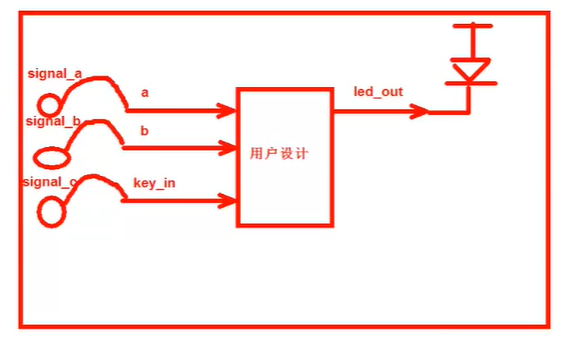``````//搭建测试平台
`timescale 1ns/1ps  // 定义仿真延时/精度，这里的 ` 为键盘左上角的

module led_test_tb;   // tb即testbench

//  激励信号定义，对应连接到待测试模块的输入端口
reg signal_a;
reg signal_b;
reg signal_c;

// 待检测信号定义，对应连接到待测试模块的输出端口
wire led;

// 例化待测试模块
led_test led_test0(
.a(signal_a),   // a 连接信号源signal_a
.b(signal_b),
.key_in(signal_c),
.led_out(led)  // 前面定义了一个led线，那么只要看led的状态就能知道led_out的状态
)；

// 产生激励，通过驱动三个激励信号源端口的状态，实现驱动待测试模块的三个端口
initial begin
signal_a = 0; signal_b = 0; signal_c = 0;
#100;  //延时100ns
signal_a = 0; signal_b = 0; signal_c = 1;
#100;
signal_a = 0; signal_b = 1; signal_c = 0;
#100;
signal_a = 0; signal_b = 1; signal_c = 1;
#100;
signal_a = 1; signal_b = 0; signal_c = 0;
#100;
signal_a = 1; signal_b = 0; signal_c = 1;
#100;
signal_a = 1; signal_b = 1; signal_c = 0;
#100;
signal_a = 1; signal_b = 1; signal_c = 1;
#200;
\$stop;   // 系统函数，停止仿真

end
``````

## 1.2 第二个栗子：3-8译码器

ABCOUT
0000000 0001
0010000 0010
0100000 0100
0110000 1000
1000001 0000
1010010 0000
1100100 0000
1111000 0000

### 新建工程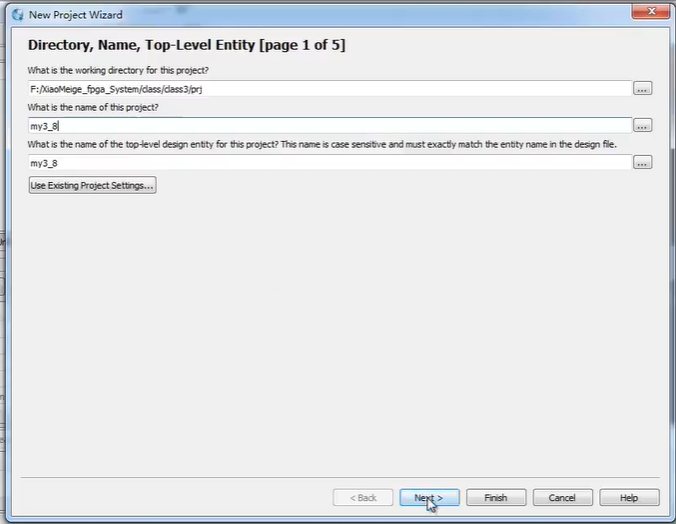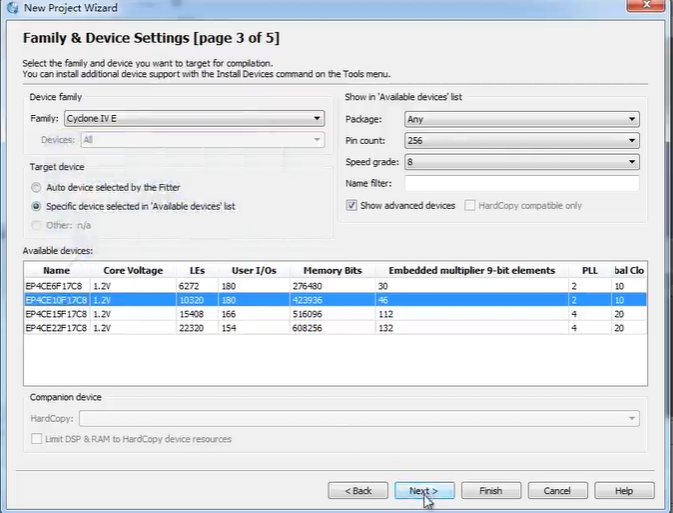### 设计输入

``````module my3_8 (a, b, c, out);

// 端口定义
input a;   // 输入端口A
input b;   //输入端口B
input c;   //输入端口C

output [7:0]out;    // 输出端口，[7:0]定义了一个多位宽的信号，高位在前，低位在后
reg [7:0] out; // 定义out 为寄存器输出型，在always中必须将out定义为 reg

// 逻辑定义
always@(a, b, c) begin // always块的敏感信号为a,b,c，当这些信号中任意一个发生变化时，就会执行always里面的内容
case({a, b, c})    // { }将a,b,c拼接成一个三位的信号
3' b000: out = 8' b0000_0001;      // 3表示信号的位宽，b表示二进制，后面的000分别对应abc
3' b001: out = 8' b0000_0010;
3' b010: out = 8' b0000_0100;
3' b011: out = 8' b0000_1000;
3' b100: out = 8' b0001_0000;
3' b101: out = 8' b0010_0000;
3' b110: out = 8' b0100_0000;
3' b111: out = 8' b1000_0000;
// 这里省略了default
endcase
end
endmodule
``````

### testbench编写

testbench 可以看作一个桌子，上面放的是待测试模块、激励信号源、信号观测示波器

``````·timescale 1ns/1ns
module my3_8_tb;

// reg 定义激励信号源
reg a;
reg b;
reg c;

// wire 输出信号的观测信号
wire [7:0] out;

//模块例化
my3_8 u1(
.a(a),
.b(b),
.c(c),
.out(out)
);
// 激励信号产生
initial begin
a = 0; b = 0; c = 0;
#200;
a = 0; b = 0; c = 1;
#200;
a = 0; b = 1; c = 0;
#200;
a = 0; b = 1; c = 1;
#200;
a = 1; b = 0; c = 0;
#200;
a = 1; b = 0; c = 1;
#200;
a = 1; b = 1; c = 0;
#200;
a = 1; b = 1; c = 1;
#200;
\$stop;

end

endmodule

``````

### 门级仿真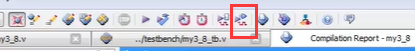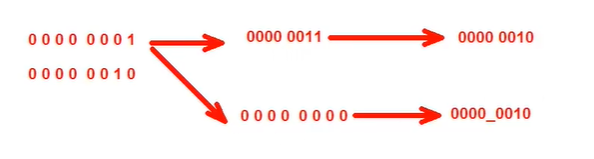# 2 层次化设计

1. 自底向上
从存在的基本单元出发，由基本单元构建高层单元，依次向上
2. 自顶向下
从系统级开始，把系统分为基本单元，在把每个单元划分位下一层次的基本单元，直到可以用EDA元件库中的元件来实现为止

暂无相关的数据...